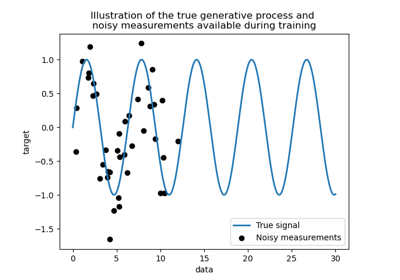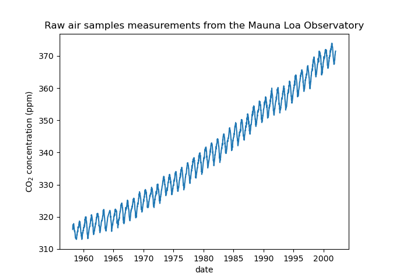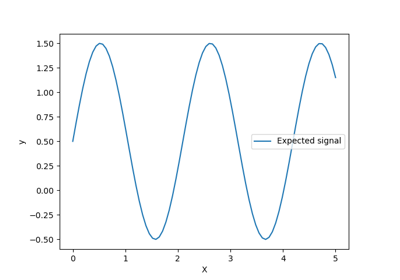# sklearn.gaussian_process.kernels.WhiteKernel¶

class sklearn.gaussian_process.kernels.WhiteKernel(noise_level=1.0, noise_level_bounds=(1e-05, 100000.0))[source]

White kernel.

The main use-case of this kernel is as part of a sum-kernel where it explains the noise of the signal as independently and identically normally-distributed. The parameter noise_level equals the variance of this noise.

$k(x_1, x_2) = noise\_level \text{ if } x_i == x_j \text{ else } 0$

Read more in the User Guide.

New in version 0.18.

Parameters:
noise_levelfloat, default=1.0

Parameter controlling the noise level (variance)

noise_level_boundspair of floats >= 0 or “fixed”, default=(1e-5, 1e5)

The lower and upper bound on ‘noise_level’. If set to “fixed”, ‘noise_level’ cannot be changed during hyperparameter tuning.

Attributes:
bounds

Returns the log-transformed bounds on the theta.

hyperparameter_noise_level
hyperparameters

Returns a list of all hyperparameter specifications.

n_dims

Returns the number of non-fixed hyperparameters of the kernel.

requires_vector_input

Whether the kernel works only on fixed-length feature vectors.

theta

Returns the (flattened, log-transformed) non-fixed hyperparameters.

Examples

>>> from sklearn.datasets import make_friedman2
>>> from sklearn.gaussian_process import GaussianProcessRegressor
>>> from sklearn.gaussian_process.kernels import DotProduct, WhiteKernel
>>> X, y = make_friedman2(n_samples=500, noise=0, random_state=0)
>>> kernel = DotProduct() + WhiteKernel(noise_level=0.5)
>>> gpr = GaussianProcessRegressor(kernel=kernel,
...         random_state=0).fit(X, y)
>>> gpr.score(X, y)
0.3680...
>>> gpr.predict(X[:2,:], return_std=True)
(array([653.0..., 592.1... ]), array([316.6..., 316.6...]))


Methods

 __call__(X[, Y, eval_gradient]) Return the kernel k(X, Y) and optionally its gradient. clone_with_theta(theta) Returns a clone of self with given hyperparameters theta. Returns the diagonal of the kernel k(X, X). get_params([deep]) Get parameters of this kernel. Returns whether the kernel is stationary. set_params(**params) Set the parameters of this kernel.

Return the kernel k(X, Y) and optionally its gradient.

Parameters:
Xarray-like of shape (n_samples_X, n_features) or list of object

Left argument of the returned kernel k(X, Y)

Yarray-like of shape (n_samples_X, n_features) or list of object, default=None

Right argument of the returned kernel k(X, Y). If None, k(X, X) is evaluated instead.

Determines whether the gradient with respect to the log of the kernel hyperparameter is computed. Only supported when Y is None.

Returns:
Kndarray of shape (n_samples_X, n_samples_Y)

Kernel k(X, Y)

K_gradientndarray of shape (n_samples_X, n_samples_X, n_dims), optional

The gradient of the kernel k(X, X) with respect to the log of the hyperparameter of the kernel. Only returned when eval_gradient is True.

property bounds

Returns the log-transformed bounds on the theta.

Returns:
boundsndarray of shape (n_dims, 2)

The log-transformed bounds on the kernel’s hyperparameters theta

clone_with_theta(theta)[source]

Returns a clone of self with given hyperparameters theta.

Parameters:
thetandarray of shape (n_dims,)

The hyperparameters

diag(X)[source]

Returns the diagonal of the kernel k(X, X).

The result of this method is identical to np.diag(self(X)); however, it can be evaluated more efficiently since only the diagonal is evaluated.

Parameters:
Xarray-like of shape (n_samples_X, n_features) or list of object

Argument to the kernel.

Returns:
K_diagndarray of shape (n_samples_X,)

Diagonal of kernel k(X, X)

get_params(deep=True)[source]

Get parameters of this kernel.

Parameters:
deepbool, default=True

If True, will return the parameters for this estimator and contained subobjects that are estimators.

Returns:
paramsdict

Parameter names mapped to their values.

property hyperparameters

Returns a list of all hyperparameter specifications.

is_stationary()[source]

Returns whether the kernel is stationary.

property n_dims

Returns the number of non-fixed hyperparameters of the kernel.

property requires_vector_input

Whether the kernel works only on fixed-length feature vectors.

set_params(**params)[source]

Set the parameters of this kernel.

The method works on simple kernels as well as on nested kernels. The latter have parameters of the form <component>__<parameter> so that it’s possible to update each component of a nested object.

Returns:
self
property theta

Returns the (flattened, log-transformed) non-fixed hyperparameters.

Note that theta are typically the log-transformed values of the kernel’s hyperparameters as this representation of the search space is more amenable for hyperparameter search, as hyperparameters like length-scales naturally live on a log-scale.

Returns:
thetandarray of shape (n_dims,)

The non-fixed, log-transformed hyperparameters of the kernel

## Examples using sklearn.gaussian_process.kernels.WhiteKernel¶Comparison of kernel ridge and Gaussian process regression

Comparison of kernel ridge and Gaussian process regressionGaussian process regression (GPR) on Mauna Loa CO2 data

Gaussian process regression (GPR) on Mauna Loa CO2 dataGaussian process regression (GPR) with noise-level estimation

Gaussian process regression (GPR) with noise-level estimation